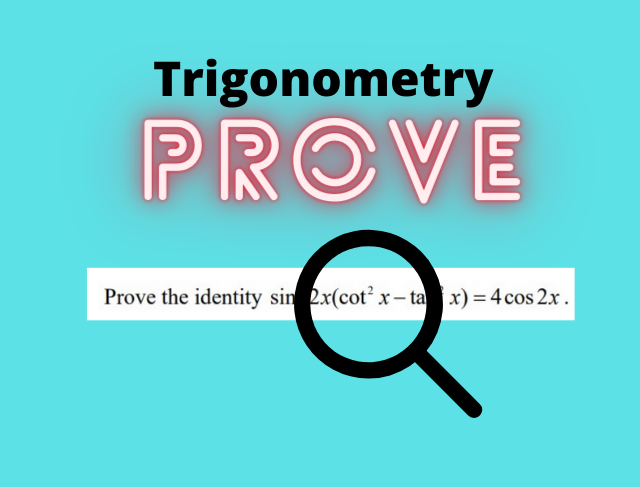# Proving Trigonometry Identity for O Level Additional Mathematics## Proving Trigonometry Identity

Proving trigonometry identity is a common type of questions often tested In O Level Additional Mathematics. To prove an identity, students are expected to use other trigonometry identities that they have learned to prove it.

The steps to prove or verify trig identities involve looking at what do you need to prove , i.e. the left hand side of the identity (LHS), and the right hand side of the identity (RHS). Next, take a look at all the mathematical formulae provided to you (the page after the cover page), and see what you should change the LHS to, to get to the RHS. After that, it involves basically manipulation of the various trigonometry functions to prove what’s on the RHS of the identity.

Of course, you can start from the right hand side (RHS) of the identity, and work towards proving that it is equal to the LHS. However, I realize that for most O Level Additional Math questions, it is usually easier to start from the LHS and move to the RHS.

## Examples of questions involving proving of trigonometry identites

Like all Math topics, the best way to master them is by practicing. Below are some examples of trigonometry questions from O Level Additional Mathematics, involving proving of the identities.

(1) By squaring sin2x + cos2x, show that sin4x + cos4x = 1 – ½sin22x.

See step- by- step worked solutions for question 1 here.

(2) Prove the identity sin 2x/ sin x – cos2x/ cos x = sec x.

See step- by- step worked solutions for question 2 here.

(3) Prove that (cos4θ-sin4θ)/(sin2θ cos2θ) =4cot2θ cosec2θ

See step- by- step worked solutions for question 3 here.

(4) Show that 1/ (cosec x – 1) – 1/ (cosec x + 1) = 2tan2x

See step- by- step worked solutions for question 4 here.

(5) Show that (2-sec2x)/(2tanx + sec2x)= (cos x – sin x)/(cos x + sin x)

See step- by- step worked solutions for question 5 here.

(6) Prove that (1 + sin 2x + cos 2x) / (cos x + sin x) = 2cos x.

See step- by- step worked solutions for question 6 here.

(7) Prove the identity sin22x (cot2x – tan2x) = 4 cos 2x.

See step- by- step worked solutions for question 7 here.

## More on trigonometry for O Level Additional Mathematics

To find out more on what’s tested in the topic of trigonometry for O Level Additional Mathematics, check out here.

For a summary of trigonometry for O Level Additional Mathematics, check out here.

error: Content is protected !!